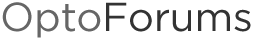# Run Hours in Pac Control

Any suggestions on making a basic hour meter within Pac Control that tracks number of hours when Variable X == 1?

‘Start timer’ when variable X = 1.

Beno,

Thank you, that was easy.

I didn’t realize until re-looking at the manual the range capability of the timer.

1 Like

Beno,

I was able to create the timer, start / pause / continue as needed per what I am trying to accomplish. However when I reset the power to the system my time is lost due to the timer resetting. Would would you suggest in order to retain the time through a power cycle and add to cumulative total run hours.

You will need to periodically store the timer value into a persistent variable - how you do this depends on how precise you need to be and how big you expect the value to reach, all depends on your specific requirements. You need to have a good understanding of the limitations of the underlying data types (storage AND arithmetic operations).

See Totalizing: 101 - On-Time totalizer pump example and read all the comments for the issues you need to consider.

If you have questions on any of that, or need a specific recommendation, please ask.

I would move the timer to a persistent variable every second or so. Keep the cumulative total also in a persistent variable.
When the controller restarts, move the timer variable back to the timer.

EDIT. @philip is a faster typist than I am.

Yep, ninja’d you there, sorry about that.

I don’t think that will do what you think it does. Up timers always start from zero.

Yup, your right, I’m wrong… Starting from zero is fine, because the cumulative timer will have the ‘master’ record in it… You don’t need to start from where you were before the power went out. (I was thinking of a down timer restarting from what ever time it had to go).

Here are the details:

I am using an (up timer / 3600) (Converting seconds to hours). If I understand it correctly per the manual the maximum up timer value is 4.611686 x 10^15 which is more than i would ever need, in addition when the power clears it will reset the up timer back to 0.

System_HourMeter = (Timer_HourMeter / 3600);

When using the above code what I am trying to accomplish work perfectly, however on a power reset the Up Timer (Timer_HourMeter) will clear thus the System_HourMeter will clear. How would I move the cumulative total into a persistent variable to not lose the cumulative total?

Is System_HourMeter an integer or a float?

System_HourMeter is Persistent Float.

``````//Do something like this one time in your power up chart
//If you don't care if you lose track of some time
//(up to 60 seconds as configured below)
//then you don't need to do this part
System_HourMeter = System_HourMeter + System_HourMeter_Temp_pstv;

//Do this in a running/looping chart somewhere
//The timer check needs to be large enough to prevent floating point addition
//failure* but small enough so your HMI shows something close to the correct
//total time hopefully those two requirements will overlap (I believe they do here),
//otherwise you will need to do something different.
if(Variable_X) then
if(Timer_HourMeter > 60.0) then //Perform addition each minute - will work for ~31years
System_HourMeter = System_HourMeter + (Timer_HourMeter/3600.0);
StartTimer(Timer_HourMeter); //Restart timer
endif
//Store current timer value in case of strategy restart
System_HourMeter_Temp_pstv = Timer_HourMeter/3600.0;
endif

//Where your variable/point turns off:
TurnOff(Variable_X);
//When your variable turns off do something like this
System_HourMeter = System_HourMeter + (Timer_HourMeter/3600.0);
StopTimer(Timer_HourMeter);
System_HourMeter_Temp_pstv = 0.0;

//*Floating points fail to add when you have a small number added to a large number
//this happens when the values are more then 2^24 apart.
//so that big_number = big_number + small_number
//it's like the addition never happened.
``````

Edit: I originally stated the above would work for 1900 years, it will actually only work for 31 years (on the accumulated value (System_HourMeter). If larger values will need to be stored, then increase the check from 60.0 to something higher (120.0 would double the amount of time).

Philip and Beno,

Thank you very much for the assistance, I see where I was going wrong, instead of taking a sample every 60 seconds I was taking it every scan.